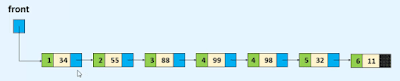A priority queue is a queue in that each element has some priority and all elements are processed based on their priorities. and the element with high priority is processed before the element with low priority.

if the two elements have the same priority then we follow the FIFO rule means the element that comes first will place first and delete first. and the element that has the highest priority is always at the front of the queue.

and in priority queue element has the highest priority has inserted first and also deleted first.Here in the above example, we implement a priority queue using a linked list. in each node, we have three parts. in the first part, we store the priority of element and in the second part we store the value of the element and in the third part, we store the linked part of the next element.

if we want to insert a new element that has priority 3 then we need to insert the element after node three.

### Program to implement priority queue using a linked list in the python programming language.

```class EmptyQueueError(Exception):
pass

class Node:

def __init__(self, value, pr):
self.info = value
self.priority = pr

class PriorityQueue:

def __init__(self):
self.front = None
def enqueue(self, data, data_priority):

temp = Node(data, data_priority)

if self.is_empty() or data_priority < self.front.priority:
self.front = temp
else:
p = self.front
while p.link != None and p.link.priority <= data_priority:

def dequeue(self):
if self.is_empty():
raise EmptyQueueError("Queue is empty")
x = self.front.info
return x

def is_empty(self):
return self.front == None
def display(self):
if self.is_empty():
print("Queue is empty")
return
print("Queue is : ")
p = self.front
while p is not None:
print(p.info, " ", p.priority)
print()

def size(self):
n = 0        p = self.front
while p is not None:
n += 1            p = p.link
return n

############################
if __name__ == '__main__':
qu = PriorityQueue()

while True:
print("1. Enqueue")
print("2. Dequeue")
print("3. Display all queue elements")
print("4. Display size of the queue")
print("5. Quit")

choice = int(input("Enter your choice : "))

if choice == 1:
x = int(input("Enter the element : "))
pr = int(input("Enter its priority : "))
qu.enqueue(x, pr)
elif choice == 2:
x = qu.dequeue()
print("Element is : ", x)
elif choice == 3:
qu.display()
elif choice == 4:
print("Size of queue ", qu.size())
elif choice == 5:
break        else:
print("Wrong choice ")
print()```